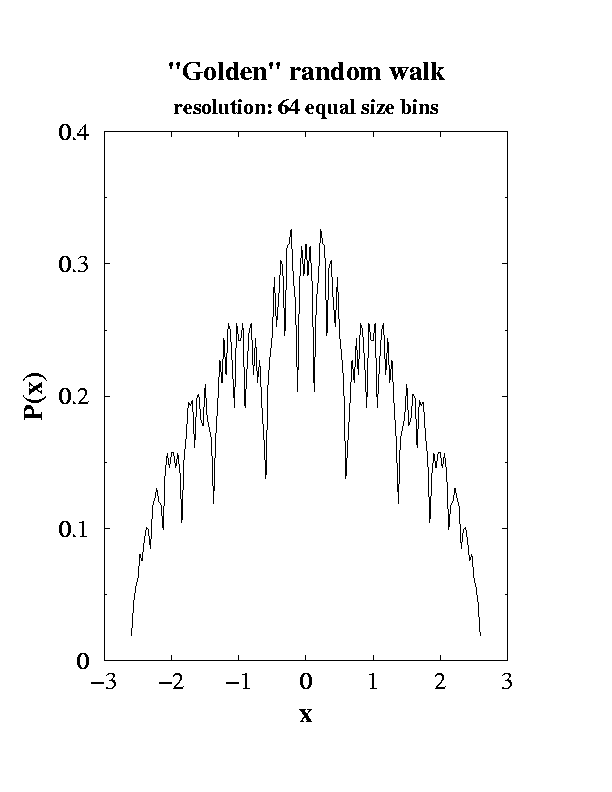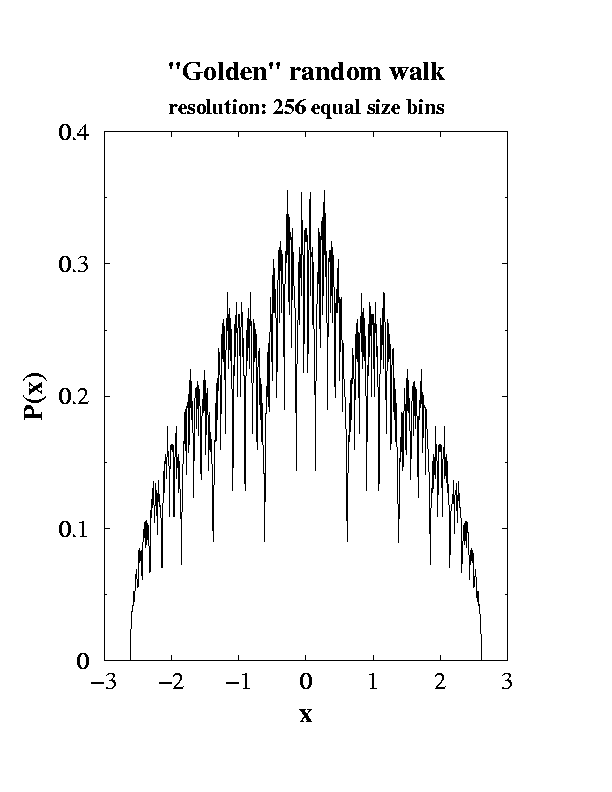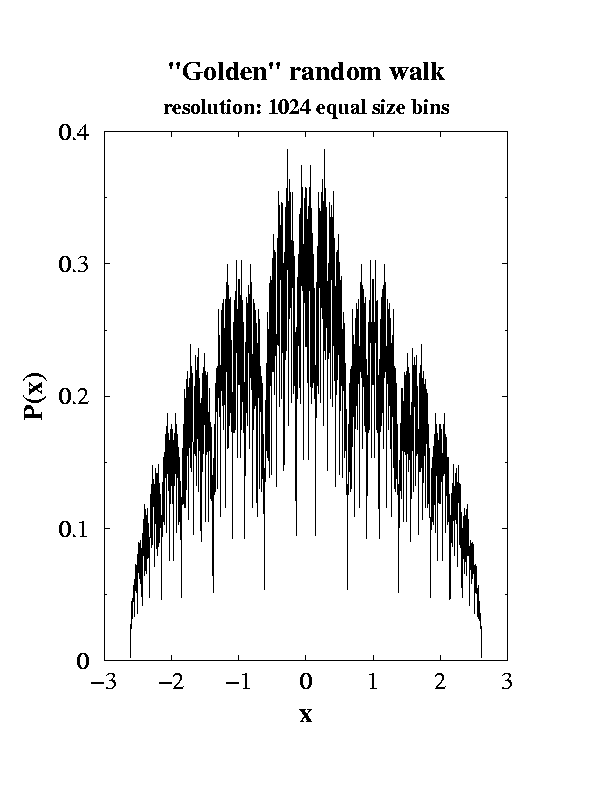## "Golden" random walk

Consider one-dimensional discrete time random walk which hops equiprobably to the right or to the left at each step, but with the length of the nth step equal to g-n, where g=(1+sqrt(5))/2 is the famous golden ratio. The probability distribution of this process is amazingly pretty, as shown in the pictures below.
The data shown are based on exact enumeration of all walks of up to 29 steps. The enumeration exploits the feature of the walk that leads to its beauty. Namely, the endpoint of an arbitrary N-step walk can be written as
x(N)= a0g-0+a1g-1+...+aNg-N
where an are randomly +1 or -1. We then use the fact that g obeys 1-g-1-g-2=0. We use this repeatedly to reduce the order of the polynomial above to first order in g. In this way, we can obtain the values x(N) for the endpoint location of each walk with perfect accuracy.Sidney Redner <redner@bu.edu>
Last modified: Sun Apr 1 09:53:19 EDT 2001